# Quick start

``````library(dyno)
library(tidyverse)``````

This tutorial quickly guides you through the main steps in the dyno workflow. For each step, we also provide a more in-depth tutorial in the user guide section.

The whole trajectory inference workflow is divided in several steps: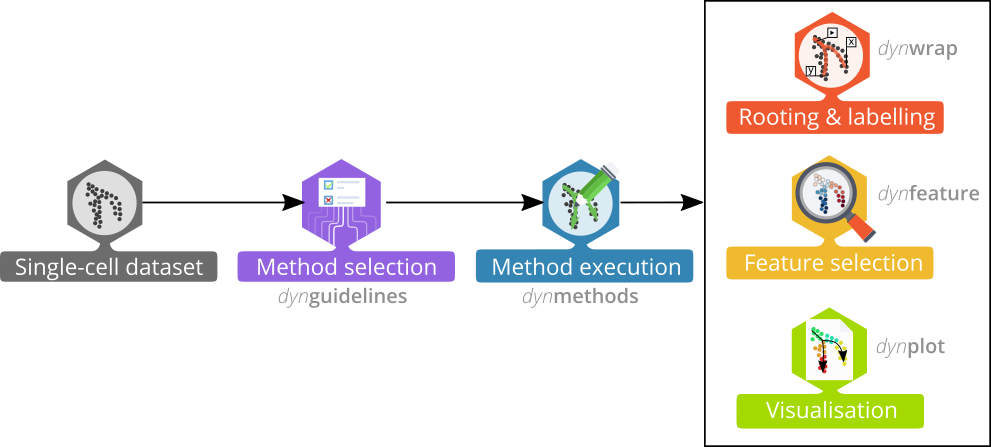## Preparing the data

The first step is to prepare the data for trajectory inference using `wrap_expression`. It requires both the counts and normalised expression (with genes/features in columns) as some TI methods are specifically built for one or the other:

``````data("fibroblast_reprogramming_treutlein")

dataset <- wrap_expression(
counts = fibroblast_reprogramming_treutlein\$counts,
expression = fibroblast_reprogramming_treutlein\$expression
)``````

➽ An in-depth tutorial for preparing your data

## Selecting the best methods for a dataset

When the data is wrapped, the most performant and scalable set of tools can be selected using a shiny app. This app will select a set of methods which are predicted to produce the most optimal output given several user-dependent factors (such as prior expectations about the topology present in the data) and dataset-dependent factors (such as the size of the dataset). This app uses the benchmarking results from dynbenchmark (doi:10.1101/276907).

``````guidelines <- guidelines_shiny(dataset)
methods_selected <- guidelines\$methods_selected``````
``````## Loading required namespace: akima
##  "slingshot" "paga_tree" "grandprix" "paga"``````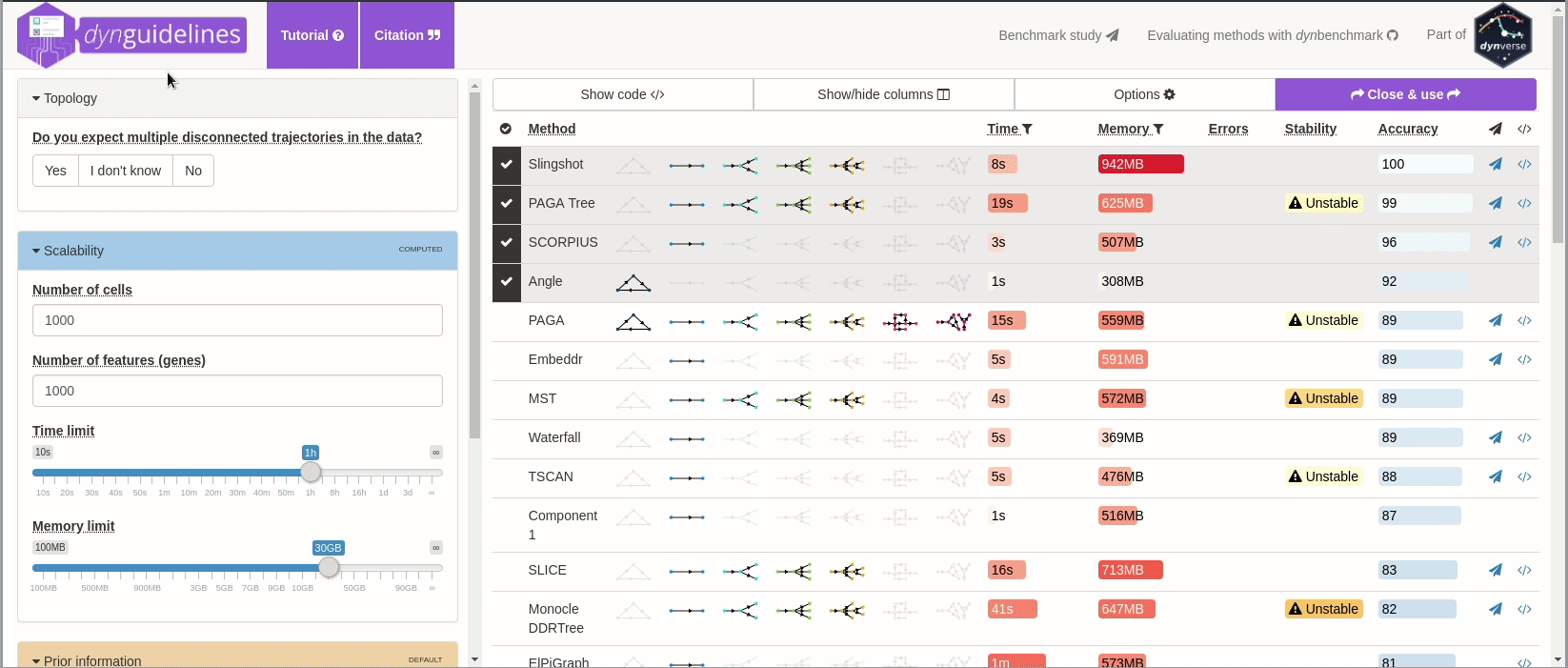➽ An in-depth tutorial for selecting the most optimal set of methods

## Running the methods

To run a method, it is currently necessary to have either docker or singularity installed. If that’s the case, running a method is a one-step-process. We will run the first selected method here:

``model <- infer_trajectory(dataset, first(methods_selected))``
``## Loading required namespace: hdf5r``

Currently, the dynmethod package contains 59 methods!

➽ An in-depth tutorial for running the methods

## Plotting the trajectory

Several visualisation methods provide ways to biologically interpret trajectories.

Examples include combining a dimensionality reduction, a trajectory model and a cell clustering:

``````model <- model %>% add_dimred(dyndimred::dimred_mds, expression_source = dataset\$expression)
plot_dimred(
model,
expression_source = dataset\$expression,
grouping = fibroblast_reprogramming_treutlein\$grouping
)
## Coloring by grouping``````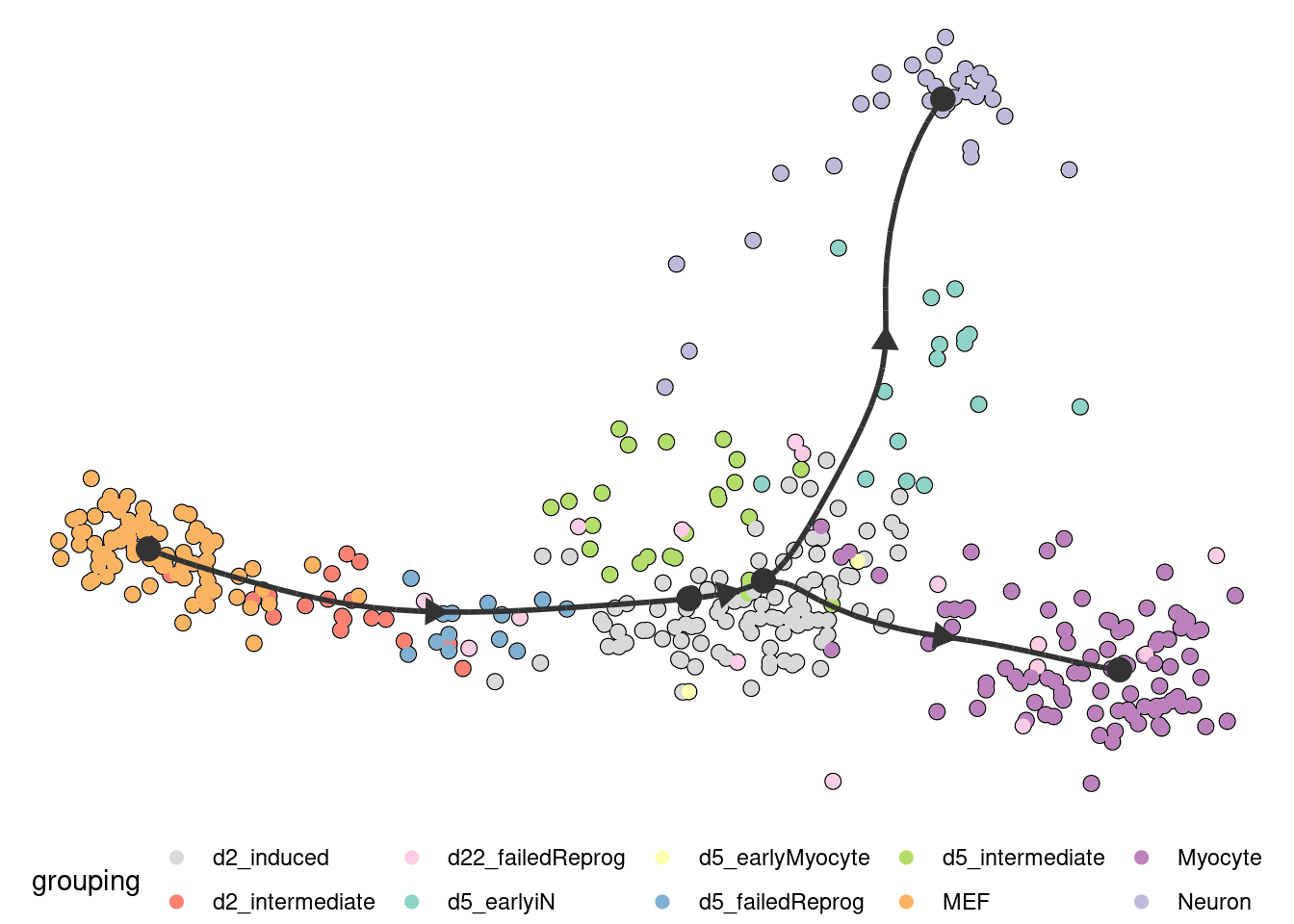Similarly, the expression of a gene:

``````plot_dimred(
model,
expression_source = dataset\$expression,
feature_oi = "Fn1"
)
## Coloring by expression``````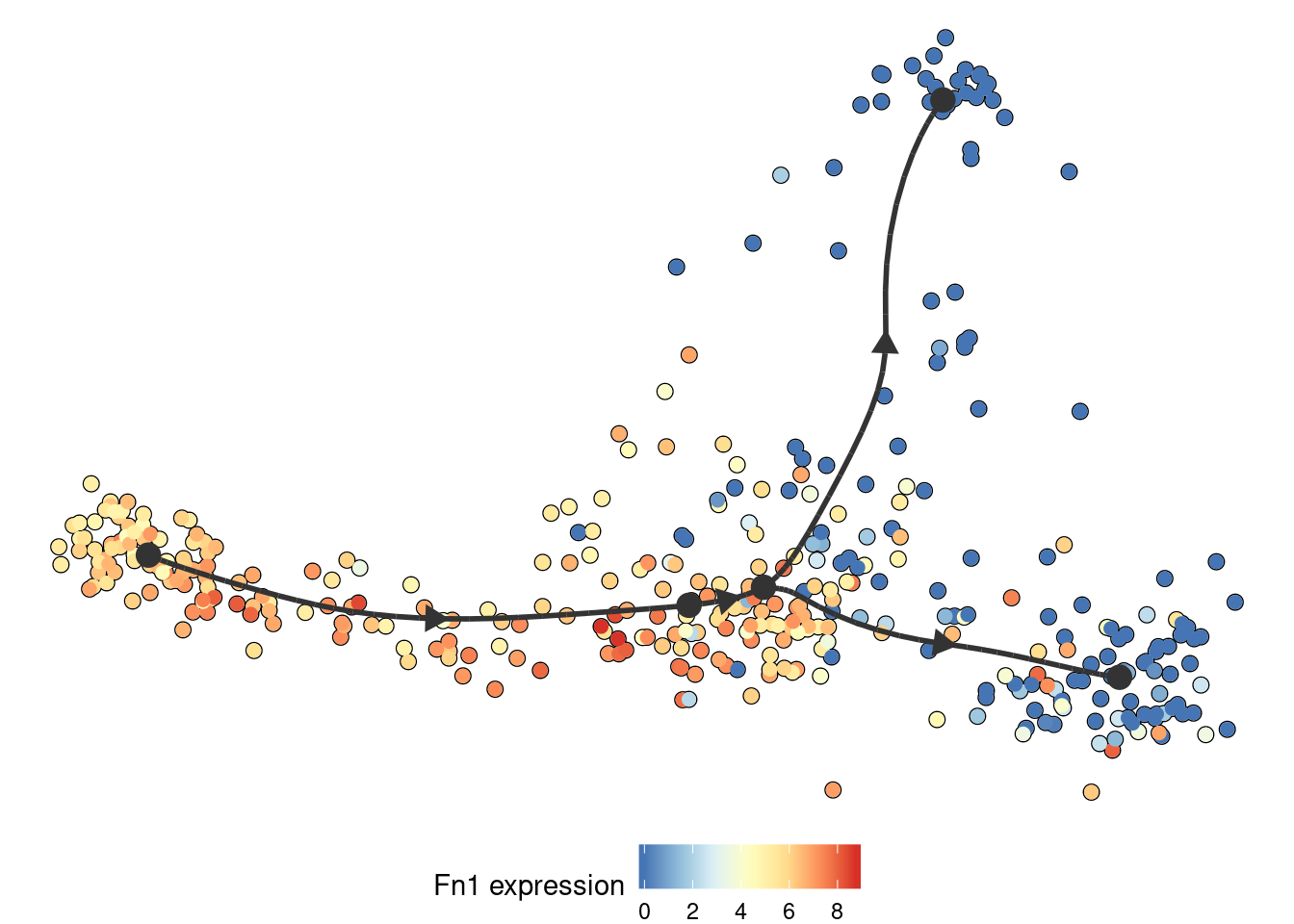Groups can also be visualised using a background color

``````plot_dimred(
model,
expression_source = dataset\$expression,
color_cells = "feature",
feature_oi = "Vim",
color_density = "grouping",
grouping = fibroblast_reprogramming_treutlein\$grouping,
label_milestones = FALSE
)``````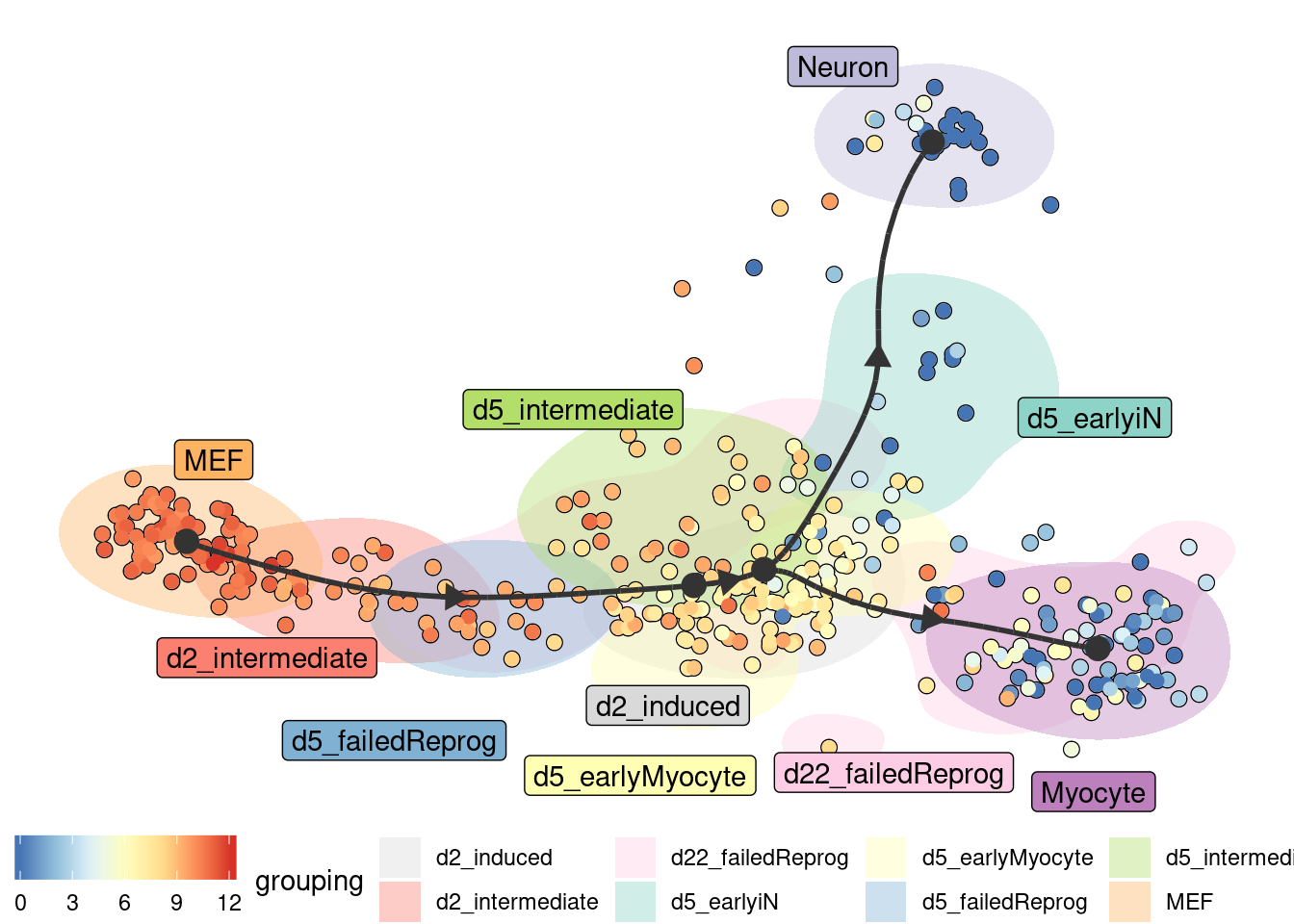➽ An in-depth tutorial for visualising the models

## Interpreting the trajectory biologically

In most cases, some knowledge is present of the different start, end or intermediary states present in the data, and this can be used to adapt the trajectory so that it is easier to interpret.

### Rooting

Most methods have no direct way of inferring the directionality of the trajectory. In this case, the trajectory should be “rooted” using some external information, for example by using a set of marker genes.

``````model <- model %>%

### Milestone labelling

Milestones can be labelled using marker genes. These labels can then be used for subsequent analyses and for visualisation.

``````model <- label_milestones_markers(
model,
markers = list(
MEF = c("Vim"),
Myocyte = c("Myl1"),
Neuron = c("Stmn3")
),
dataset\$expression
)``````

➽ An in-depth tutorial for adapting the trajectory

## Predicting and visualising genes of interest

We integrate several methods to extract candidate marker genes/features from a trajectory.

### A global overview of the most predictive genes

At default, the overall most important genes are calculated when plotting a heatmap.

``````plot_heatmap(
model,
expression_source = dataset\$expression,
grouping = fibroblast_reprogramming_treutlein\$grouping,
features_oi = 50
)
## No features of interest provided, selecting the top 50 features automatically
## Using dynfeature for selecting the top 50 features
## Coloring by grouping``````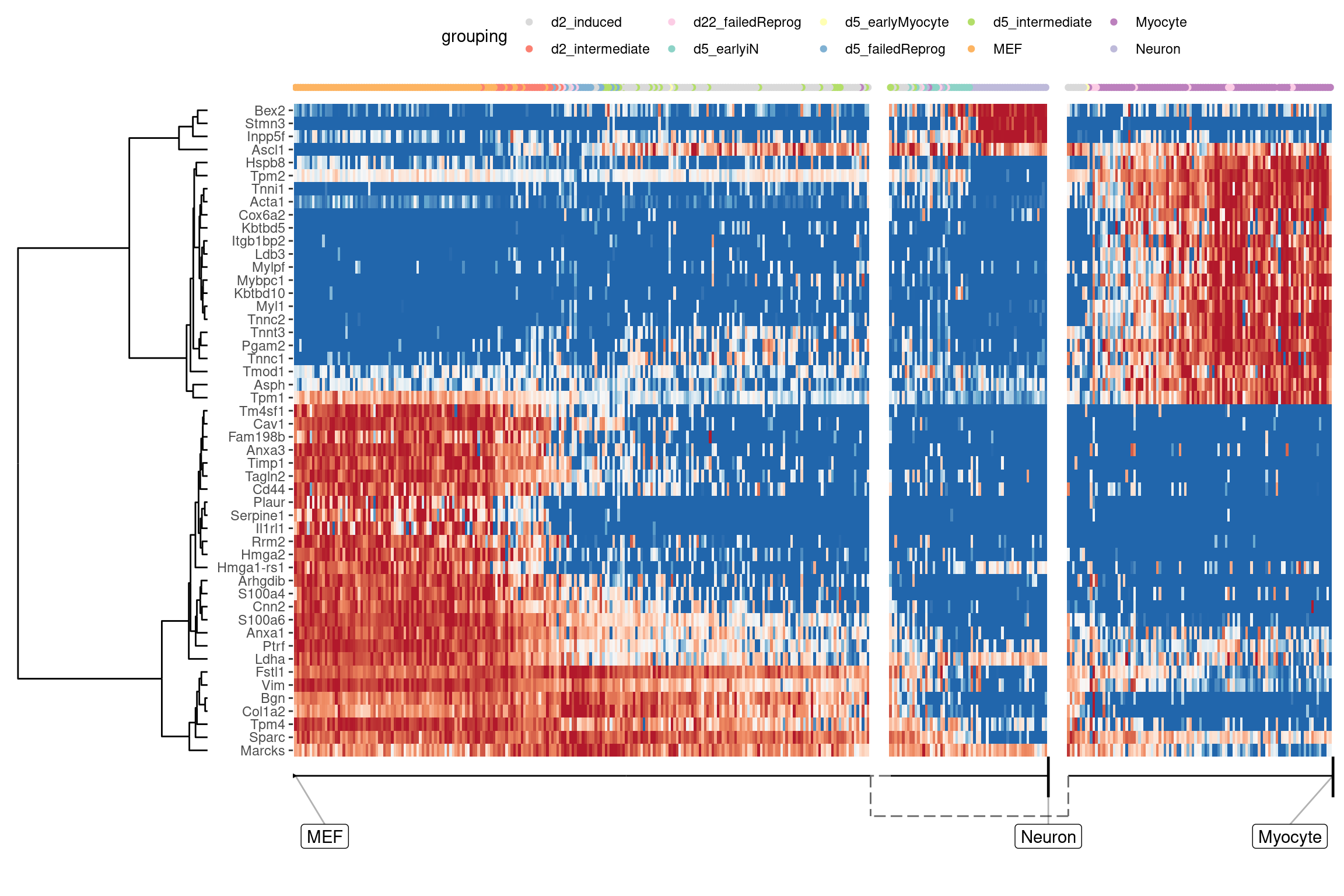### Lineage/branch markers

We can also extract features specific for a branch, eg. genes which change when a cell differentiates into a Neuron

``````branch_feature_importance <- calculate_branch_feature_importance(model, expression_source=dataset\$expression)

neuron_features <- branch_feature_importance %>%
filter(to == names(model\$milestone_labelling)[which(model\$milestone_labelling =="Neuron")]) %>%
top_n(50, importance) %>%
pull(feature_id)``````
``````plot_heatmap(
model,
expression_source = dataset\$expression,
features_oi = neuron_features
)
## Coloring by milestone``````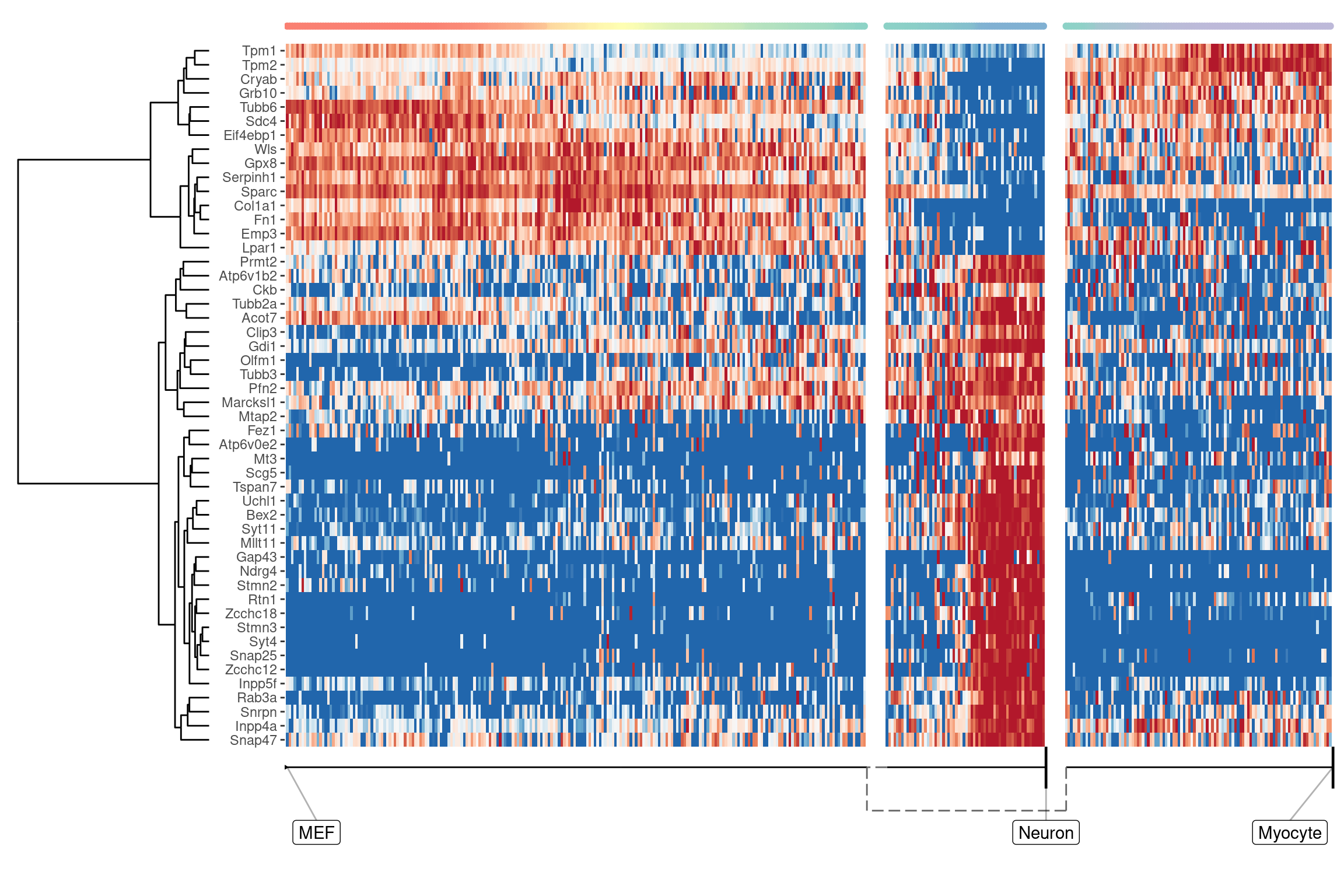### Genes important at bifurcation points

We can also extract features which change at the branching point

``````branching_milestone <- model\$milestone_network %>% group_by(from) %>% filter(n() > 1) %>% pull(from) %>% first()

branch_feature_importance <- calculate_branching_point_feature_importance(model, expression_source=dataset\$expression, milestones_oi = branching_milestone)

branching_point_features <- branch_feature_importance %>% top_n(20, importance) %>% pull(feature_id)

plot_heatmap(
model,
expression_source = dataset\$expression,
features_oi = branching_point_features
)
## Coloring by milestone``````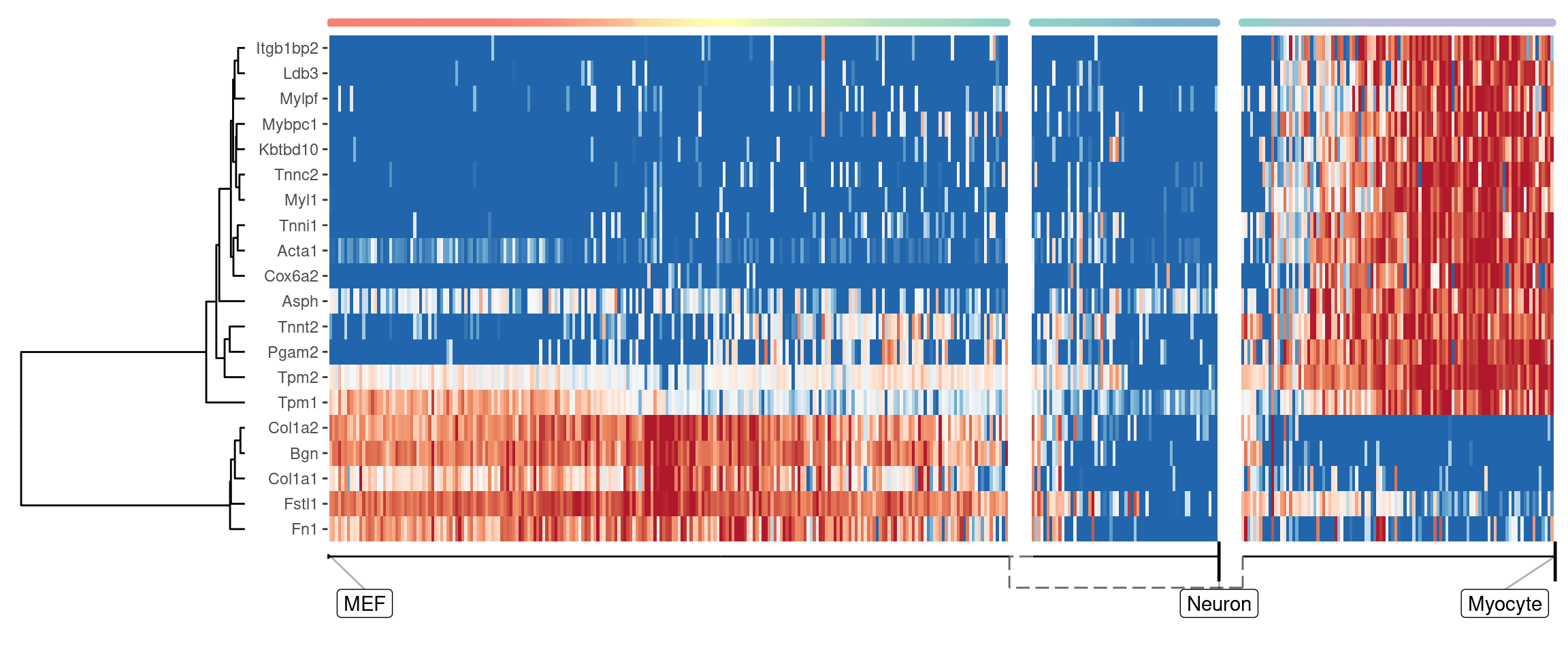``````space <- dyndimred::dimred_mds(dataset\$expression)

map(branching_point_features[1:12], function(feature_oi) {
plot_dimred(model, dimred = space, expression_source = dataset\$expression, feature_oi = feature_oi, label_milestones = FALSE) +
theme(legend.position = "none") +
ggtitle(feature_oi)
}) %>% patchwork::wrap_plots()``````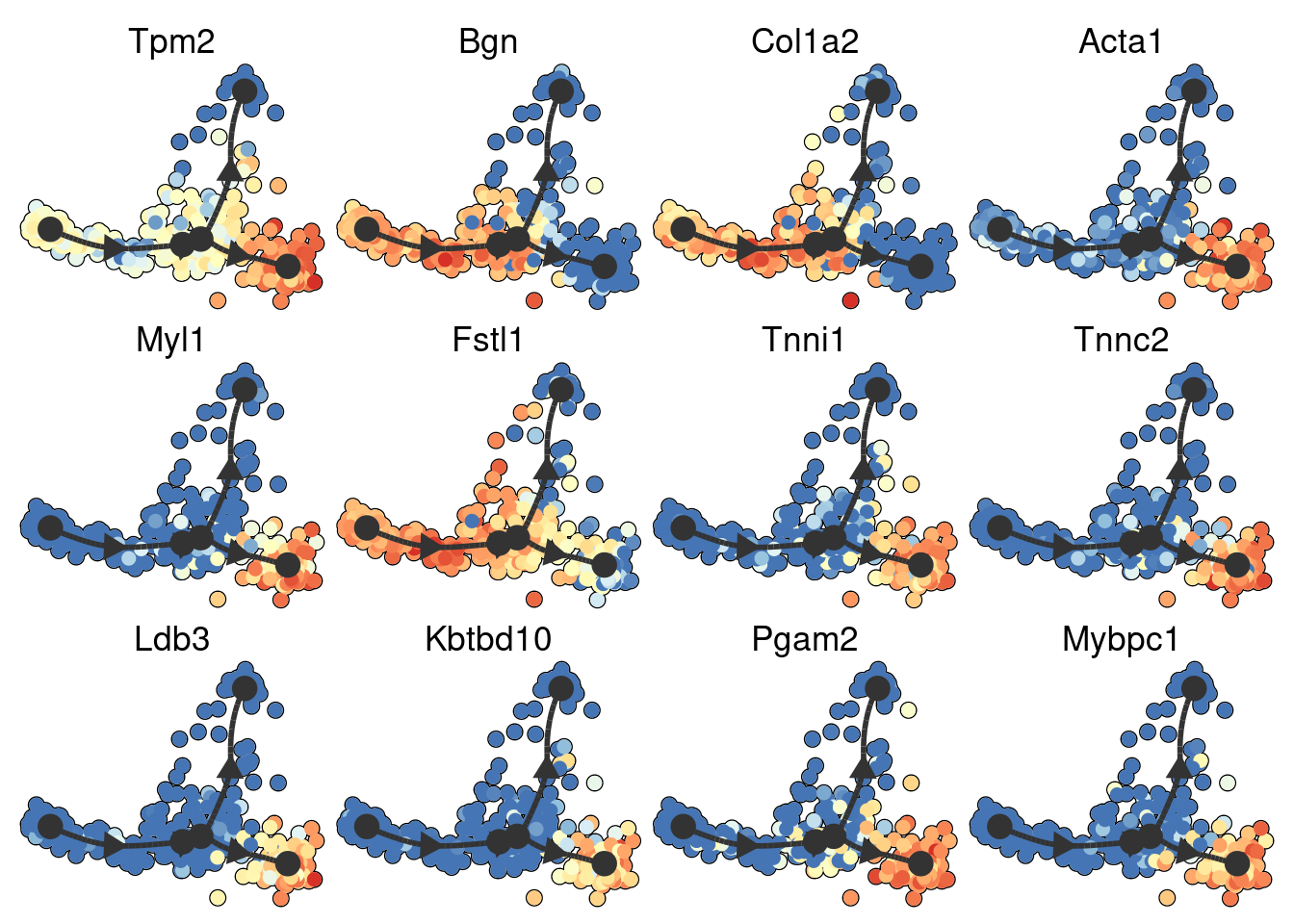➽ An in-depth tutorial for finding trajectory differentially expressed genes